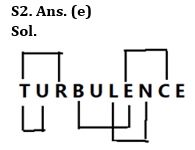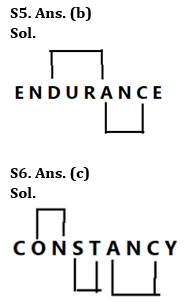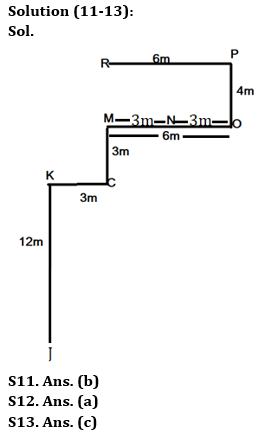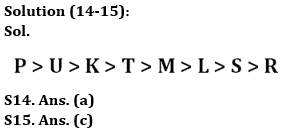Latest Banking jobs   »

# Reasoning Ability Quiz For Bank Foundation 2023 -16th April

Q1. If it is possible to make a meaningful word from 1st, 2nd, 4th, 5th and 6th letters of word “EMPIRICAL”, then which will be the 2nd letter of the word from left end? If no such word can be formed, then mark the answer as ‘Y’. If more than one word can be formed mark the answer as ‘X’.
(a) Y
(b) M
(c) E
(d) I
(e) X

Q2. How many pairs of letters are there in the word “TURBULENCE” which has as many letters between them in the word as in the alphabetical series (Both forward and backward direction)?
(a) One
(b) Two
(c) Three
(d) Four
(e) More than four

Q3. If all the even digits of the number “564854538” are decreased by ‘1’ and then all the digits are arranged in ascending order from left to right, then what will be the sum of fifth digit from left end and third digit from the right end?
(a) 3
(b) 4
(c) 6
(d) 8
(e) None of these

Q4. If all the consonants of the Word “ACTUATE” are replaced by immediately preceding letter & vowels to its immediately following letter in the alphabetical series, how many vowels are there in the rearranged word?
(a) Two
(b) Three
(c) Four
(d) Five
(e) None

Q5. How many pairs of letters are there in the word “ENDURANCE” which has as many letters between them in the word as in the alphabetical series (Both forward and backward direction)?
(a) One
(b) Two
(c) Three
(d) Four
(e) More than four

Q6. How many pairs of letters are there in the word “CONSTANCY” which has as many letters between them in the word as in the alphabetical series (Both forward and backward direction)?
(a) One
(b) Two
(c) Three
(d) Four
(e) More than four

Q7. If all the odd digits of the number “746587835” are increased by ‘1’ and then all the digits are arranged in descending order from left to right, then what will be the difference of fifth digit from left end and third digit from the right end?
(a) 3
(b) 4
(c) 0
(d) 6
(e) None of these

Q8. Which of the following letter will be third letter from the right end if the letters at odd positions are replaced by just succeeding letter in the word “NATIONAL”?
(a) P
(b) N
(c) B
(d) L
(e) None of the above

Q9. If it is possible to make a meaningful word from 1st, 3rd, 5th and 6th letters of word “SUBSPECIES”, then which will be the 3rd letter of the word from left end? If no such word can be formed, then mark the answer as ‘N’. If more than one word can be formed mark the answer as ‘M’.
(a) N
(b) M
(c) E
(d) S
(e) B

Q10. If it is possible to make a meaningful word from 2nd, 5th, 7th and 11th letters of word “APPROPRIATE”, then which will be the 1st letter of the word from left end? If no such word can be formed, then mark the answer as ‘Y’. If more than one word can be formed mark the answer as ‘X’.
(a) Y
(b) P
(c) O
(d) R
(e) X

Directions (11-13): Answer the questions based on the information given below.
Nine friends J, K, L, M, N, O, P, Q & R sit in a park in a certain arrangement. J sits 12m south of K. O sits 6m east of M. C sits 3m east of K. M sits 3m north of C. N sits exactly in the middle of M and O. P sits 4m north of O. R sits 6m west of P.

Q11. What is the direction of J with respect to R?
(a) North-west
(b) South- west
(c) North- east
(d) South- east
(e) None of the above

Q12. What is the shortest distance between N and P?
(a) 5m
(b) 6m
(c) 7m
(d) 8m
(e) None of the above

Q13. What is the direction of P with respect to K?
(a) North-west
(b) South- west
(c) North- east
(d) South- east
(e) None of the above

Directions (14-15): answer the questions based on the information given below.

Eight persons have different amount of money with them. K has more money than T who has more money than M and L. L has more money than S but lesser money than M. Only P has more money than U. R has less money than U. M has more money than S who has second least amount of money.

Q14. Who has 3rd least amount of money?
(a) L
(b) R
(c) T
(d) M
(e) None of the above

Q15. How many persons have less money than K?
(a) Three
(b) Four
(c) Five
(d) Two
(e) None of these

Solutions

S1. Ans. (a)
Sol.
1st, 2nd, 4th, 5th and 6th letters of word – E, M, I, R, I
Hence, no meaningful word can be formed. So, Y is the correct answer.S3. Ans. (e)
Sol. Given number – 564854538
After operation – 553753537
Ascending order – 333555577
So, required sum = 5 +5 = 10

S4. Ans. (e)
Sol. Consonants are replaced by its previous letter and vowels to its next letter i.e. BBSVBSF. So, there is no vowel in rearranged wordS7. Ans. (c)
Sol. Given number – 746587835
After operation – 846688846
Descending order – 888866644
Required difference = 6 – 6 = 0

S8. Ans. (b)
Sol. If we replaced odd positioned letters with their just succeeding letter, then we would get the respective word “OAUIPNBL”. So, the third letter from right end is N.

S9. Ans. (a)
Sol. 1st, 3rd, 5th and 6th letters of word – S, B, P, E
Hence, no meaningful word can be formed.

S10. Ans. (e)
Sol. 2nd, 5th, 6th and 7th letters of word – P, O, R, E
So, three words can be formed – PORE, ROPE, REPO. Hence, X is the correct answer.## FAQs

### What is the selection process of the Bank Clerk?

The selection process of the Bank Clerk is Prelims & Mains.

#### Congratulations!Union Budget 2023-24: Free PDF